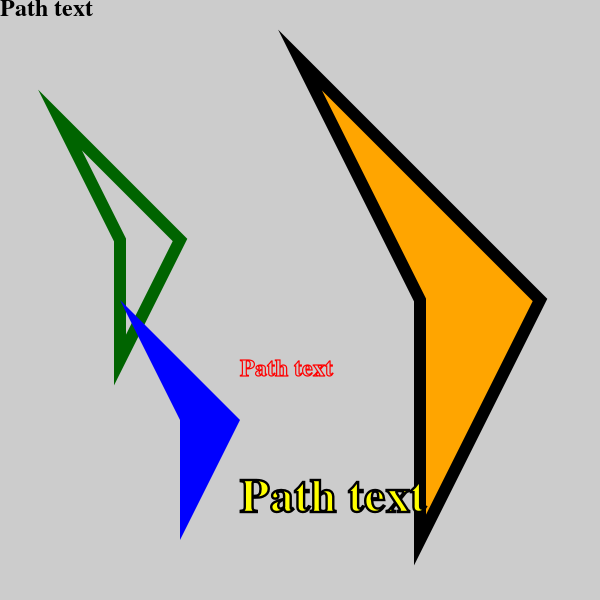# Path objects in generativepy

Martin McBride, 2020-10-04
Tags generativepy tutorial path
Categories generativepy generativepy tutorialThis tutorial covers the basics of using Path objects in generativepy.

You can think of a `Path` as being an abstract shape:

• You can create a `Path` object in the same way as you would draw a shape, but creating the `Path` doesn't draw anything.
• You can then draw the path as many times as you want, using `fill` and/or `stroke`.
• You can also create a clipping region using the path.

You can draw the same path in different ways (eg different fill or stroke styles), in different positions, or even scaled and rotated.

## Path example code

Here is a sample Python program for creating various filled and outlined rectangles. The code is explained later in this article:

```from generativepy.drawing import make_image, setup
from generativepy.color import Color
from generativepy.geometry import Path, Polygon, Text
import math

def draw(ctx, width, height, frame_no, frame_count):
setup(ctx, width, height, width=5, background=Color(0.8))

# Get a polygon path object
path1 = Polygon(ctx).of_points([(0, 0), (1, 1),
(0.5, 2), (0.5, 1)])\
.path()

# Get a text path object
path2 = Text(ctx).of("Path text", (0, 0))\
.font("Times")\
.size(0.2)\
.align_left()\
.align_top()\
.path()

# Apply the polygon in various places
ctx.save()
ctx.translate(0.5, 1)
Path(ctx).of(path1).stroke(Color('darkgreen'), 0.1)
ctx.restore()

ctx.save()
ctx.translate(1, 2.5)
Path(ctx).of(path1).fill(Color('blue'))
ctx.restore()

ctx.save()
ctx.translate(2.5, 0.5)
ctx.scale(2, 2)
Path(ctx).of(path1).fill(Color('orange')).stroke(Color('black'), 0.05)
ctx.restore()

# Apply the text in various places
ctx.save()
ctx.translate(0, 0)
Path(ctx).of(path2).fill(Color('black'))
ctx.restore()

ctx.save()
ctx.translate(2, 3)
Path(ctx).of(path2).stroke(Color('red'), 0.01)
ctx.restore()

ctx.save()
ctx.translate(2, 4)
ctx.scale(2, 2)
Path(ctx).of(path2).fill(Color('yellow')).stroke(Color('black'), 0.01)
ctx.restore()

make_image("path-method.png", draw, 600, 600)
```

Here is the resulting image:## Creating the paths

The first part of the code creates a couple of paths. Here is the first:

```    path1 = Polygon(ctx).of_points([(0, 0), (1, 1),
(0.5, 2), (0.5, 1)])\
.path()
```

In this case, we have created a `Polygon` in the usual way, but instead of filling or stroking the shape, we call `path`. This returns a Pycario path object. You can think of the path object as being like a set of instructions for drawing the shape.

This code doesn't draw anything, it just saves the drawing instructions in `path1`.

Here is the second path:

```    path2 = Text(ctx).of("Path text", (0, 0))\
.font("Times")\
.size(0.2)\
.align_left()\
.align_top()\
.path()
```

This time we have created a complex path - some text. But the result is the same, we don't draw anything but the instructions for drawing the text are store in `path2`.

## Drawing path1

Here is the code that actually draws `path1`

```    ctx.save()
ctx.translate(0.5, 1)
Path(ctx).of(path1).stroke(Color('darkgreen'), 0.1)
ctx.restore()

ctx.save()
ctx.translate(1, 2.5)
Path(ctx).of(path1).fill(Color('blue'))
ctx.restore()

ctx.save()
ctx.translate(2.5, 0.5)
ctx.scale(2, 2)
Path(ctx).of(path1).fill(Color('orange')).stroke(Color('black'), 0.05)
ctx.restore()
```

In the first section, we save the current context and then call `translate`. This moves user space by `(0.5, 1)`. See useful context methods for more information about this.

We then use a `Path` object. This takes the drawing instructions in `path1` and adds them to the context ready to be drawn. We then stroke the shape in dark green. If you look at the definition of the polygon that is used to define `path1`, you will see that the top left corner of the polygon is at `(0, 0)`, but because we have translated user space, the polygon actually gets drawn at `(0.5, 1)`.

Finally, we call `restore`. This sets the context back to the state it was in when `save` was called, so it effectively undoes the translation.

The second block draws the same shape again, this time at `(1, 2.5)`, and it fills it rather than outlining it.

The third block draws the shape yet again, this time at `(2.5, 0.5)`. In this case, we also scale user space by `(2, 2)`, which scales user space in the x and y directions. We fill and outline the shape, and because of the scaling, the shape appears twice as big.

As we said before, `restore` takes us back to the previous `save`, so in this case, it undoes the translate and the scale.

## Drawing path2

Here is the code that draws `path2`

```    ctx.save()
ctx.translate(0, 0)
Path(ctx).of(path2).fill(Color('black'))
ctx.restore()

ctx.save()
ctx.translate(2, 3)
Path(ctx).of(path2).stroke(Color('red'), 0.01)
ctx.restore()

ctx.save()
ctx.translate(2, 4)
ctx.scale(2, 2)
Path(ctx).of(path2).fill(Color('yellow')).stroke(Color('black'), 0.01)
ctx.restore()
```

This illustrates the use of complex paths. We draw the same text path filled, stroked, and also scaled, filled and stroked.

If you found this article useful, you might be interested in the book Computer Graphics in Python or other books by the same author.

#### Popular tags

2d arrays abstract data type alignment and animation arc array arrays behavioural pattern bezier curve built-in function callable object circle classes close closure cmyk colour comparison operator comprehension context context manager conversion creational pattern data types design pattern device space dictionary drawing duck typing efficiency else encryption enumerate fill filter font font style for loop function function composition function plot functools game development generativepy tutorial generator geometry gif gradient greyscale higher order function hsl html image image processing imagesurface immutable object index inner function input installing iter iterable iterator itertools l system lambda function len line linspace list list comprehension logical operator lru_cache magic method mandelbrot mandelbrot set map monad mutability named parameter numeric python numpy object open operator optional parameter or partial application path polygon positional parameter print pure function pycairo radial gradient range recipes rectangle recursion reduce rgb rotation scaling sector segment sequence singleton slice slicing sound spirograph sprite square str strategy stream string stroke structural pattern subpath symmetric encryption template text text metrics tinkerbell fractal transform translation transparency tuple turtle unpacking user space vectorisation webserver website while loop zip